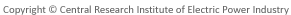#※ PDFのファイルサイズが大きい場合には、ダウンロードに時間がかかる場合がございます。　ダウンロードは1回のクリックで開始しますので、ダウンロードが完了するまで、複数回のクリックはなさらないようご注意願います。

## 電力中央研究所　報告書（電力中央研究所報告）

### 報告書データベース　詳細情報

V08064

#### タイトル（英文）

Experiments on sediment transport rate due to tsunami and proposal of pickup rate formula of suspended sand

#### 概要 （英文）

Sediment transport experiment due to tsunami was performed using a wave flume with a piston type wave paddle. Horizontal fine sand layer was setup with the length of 10.5m. Still water depth was 9cm on the sand layer. The range of the Shield's number in this experiment corresponded to 0-1. The grain sizes of sand used were 0.08mm and 0.2mm. Generating one solitary wave with the wave height of 3 to 6 cm and the run-up time of 30s and without wave breaking, water surface elevation, suspended concentration and velocity were measured on the horizontal sand layer. The vertical distributions of them were obtained in detail. Sediment transport amount corresponding to one wave was also caught in the trap set up at the end of sand layer. Sediment transport rate passing through the total water depth and bed load transport rate passing near the seabed were measured. Pickup rate of seabed sand was estimated from the balance of suspended sediment flux. The conventional pickup rate formula using the Shield's number alone as a parameter included the factor depending on the sand grain size. So, the property of the no-dimensional pickup rate estimated by the conventional formula was significantly affected by the sand grain size. Using the dimensional analysis, new two no-dimensional dominant parameters such as 'no-dimensional sand grain diameter' and 'no-dimensional settling velocity', were introduced. And a new pickup rate formula of suspended sand was proposed by using their two parameters and the Shield's number. Generally, the new pickup rate formula could reproduce the experimental results of two sand grain sizes, and pickup rates of the recent tsunami experiment (Takahashi et al., 1999) better than the conventional formula. On the other hand, the bed load transport rate formula by Ashida and Michiue could well reproduce the bed load transport rate by the above trap experiments. So, we recommended the combination of the new pickup rate formula and the bed load transport rate formula by Ashida and Michiue as the sediment transport rate formula for tsunami. A seabed deformation numerical model using the above sediment transport rate formula was applied to the physical model test on seabed deformation around a harbor due to tsunami. It was showed that the above formula was able to reproduce the experimental seabed deformation better than the conventional formula.

2008

2009/07

#### キーワード